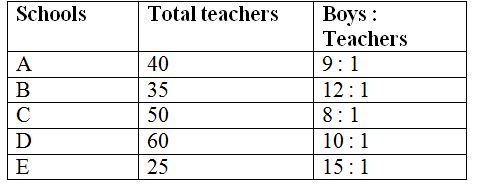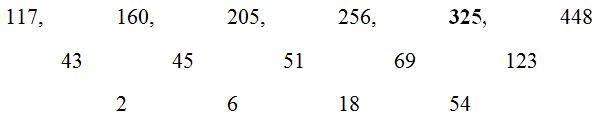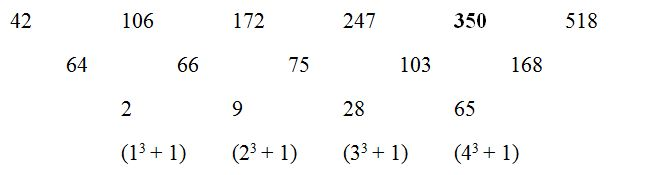# SBI Clerk/EPFO Assistant Prelims Quantitative Aptitude Questions 2019 (Day-11)

Quantitative aptitude is one of the most focusing subjects among banking aspirants.In SBI Clerk/EPFO Assistant Prelims there are 35 Marks allotted for this section. The Marks itself shows how weightage has given for Quantitative aptitude section. The SBI Clerk /EPFO Assistant 2019 exam is approaching very shortly. The exam tentatively scheduled in June 2019. Understand the importance of time and use it in the right way. As we know that SBI aptitude test questions are easy but difficult to solve within the given time limit. So practice the only solution to perform well at the time of examination. Keeping all the things in mind we are giving attention for SBI Clerk Prelims Quantitative Aptitude section. Here you can get SBI Clerk/EPFO Assistant Prelims Quantitative Aptitude Questions and answer on a daily basis. SBI Clerk Prelims Quantitative Aptitude Quiz will enhance your sped and accuracy in a self-directed way.

[WpProQuiz 6471]

Directions (Q. 1 – 5): Find the wrong term in the following number series?

1) 117, 160, 205, 256, 327, 448

a) 327

b) 205

c) 160

d) 448

e) 256

2) 2, 26, 286, 2004, 10010, 30030

a) 286

b) 10010

c) 30030

d) 2004

e) 26

3) 187, 94, 95, 192, 778, 6216

a) 6216

b) 778

c) 94

d) 95

e) 192

4) 4, 8, 25, 91, 387, 1981

a) 91

b) 25

c) 387

d) 1981

e) 8

5) 42, 106, 172, 247, 351, 518

a) 518

b) 247

c) 172

d) 106

e) 351

Directions (Q. 6 – 10): Study the following information carefully and answer the given questions.

The following table shows the total number of teachers in 5 different schools and the ratio of boys to that of teachers in those schools.6) Find the average number of boys in the schools A, B and D together?

a) 420

b) 445

c) 475

d) 460

e) None of these

7) Total number of boys in the school C is approximately what percentage of total number of boys in the school E?

a) 124 %

b) 107 %

c) 143 %

d) 92 %

e) 75 %

8) Find the total number of girls in the school A and C together, if the total number of students in school A is 800 and the ratio of boys to that of girls in the school C is 5: 4?

a) 840

b) 720

c) 760

d) 880

e) None of these

9) Find the difference between the average number of boys in School A and D together to that of the school B and C together?

a) 70

b) 90

c) 80

d) 60

e) None of these

10) Find the total number of students in school D, if the total number of students in school E is 700 and the total number of girls in school D is 225 more than the total number of girls in School E?

a) 1450

b) 1300

c) 900

d) 1150

e) None of theseThe difference of difference is, *3

The wrong term is, 327

2 * 13 = 26

26 * 11 = 286

286 * 7 = 2002

2002 * 5 = 10010

10010 * 3 = 30030

The wrong term is, 2004

187 * 0.5 + 0.5 = 94

94 * 1 + 1 = 95

95 * 2 + 2 = 192

192 * 4 + 4 = 776

776 * 8 + 8 = 6216

The wrong term is, 778

4 * 1 + 22 = 8

8 * 2 + 32 = 25

25 * 3 + 42 = 91

91 * 4 + 52 = 389

389 * 5 + 62 = 1981

The wrong term is, 387The wrong term is, 351

The average number of boys in the schools A, B and D together

= > [(9 * 40) + (12 * 35) + (10 * 60)]/3

= > [360 + 420 + 600]/3 = 460

Total number of boys in the school C

= > (8 * 50) = 400

Total number of boys in the school E

= > (15 * 25) = 375

Required % = (400/375) * 100 = 106.667 % = 107 %

The total number of students in the school A = 800

Total number of boys in school A = 9 * 40 = 360

Total number of girls in school A = 800 – 360 = 440

The ratio of boys to that of girls in the school C = 5 : 4

Total number of boys in school C = 8 * 50 = 400

Total number of girls in school C = (400/5) * 4 = 320

Required total = 440 + 320 = 760

The average number of boys in School A and D together

= > [(9 * 40) + (10 * 60)]/2

= > [360 + 600]/2 = 480

The average number of boys in School B and C together

= > [(12 * 35) + (8 * 50)]/2

= > [420 + 400]/2 = 410

Required difference = 480 – 410 = 70

The total number of students in school E = 700

Total number of boys in School E = (15 * 25) = 375

Total number of girls in School E = 700 – 375 = 325

Total number of boys in School D = (10 * 60) = 600

Total number of girls in School D = 325 + 225 = 550

The total number of students in school D = 600 + 550 = 1150

3 1 vote
Rating